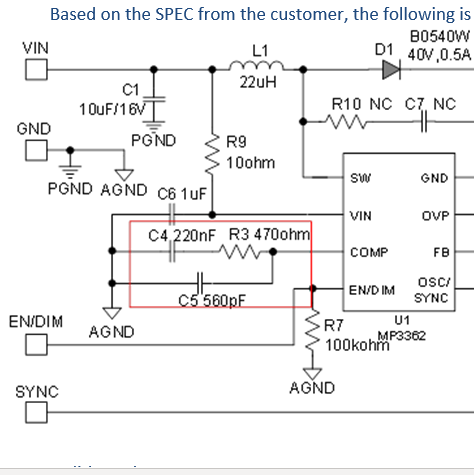# MP(Q)3362 - GCS information is missing?

Hello,
in the datasheet there is an explanation on how to do the dimension of the compensation network (site 13/14). To use equation 16 there is G_CS needed - the conductance of the current sensing circuit. The current sensing circuit is meant to be the resistor serial to the transistor in the block diagramm (?). In the electrical characteristics there is only the SW on resistance 80mOhm which can be translated to G_CS but this would also include R_DSon of the transistor? What is the correct value of GCS?

Hi jchlond,

Thanks for your question. I’m looking into this.

Cindy

Hi,

Can you provide the spec details you are using with this part, Vin, Vout, Iout?
We need to do a bench test to suggest the recommended COMP resistance.

Thanks,
Cindy

Hi,

Vin = 5V
Vout = 16V
Iout = 40mA

Johannes

Hi Johannes,

From your provided parameters, the following is the suggested component selection on the COMP pin.Also, the inverse of R_ON is the conductance of the internal current sense circuit.

Thanks,
Cindy

Hi Cindy,
thanks for the effort. I forgot two things to mention.

We use another frequency. In our case it´s 1,025MHz (resistor at OSC/SYNCH 39kOhm).
Our inductance is 47µH.

In this case we need another dimension for the compensation network. Also it would be good to know what kind of tool you used to calculate the compensation network as with the datasheet alone it was not possible for us to do so.

Thanks,
Johannes